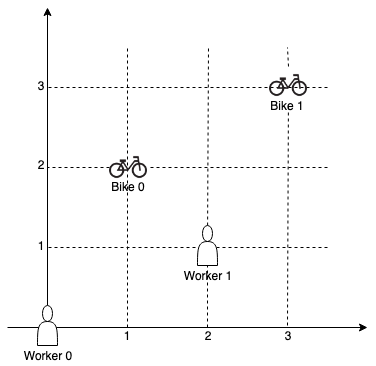1066. Campus Bikes II

On a campus represented as a 2D grid, there are `N` workers and `M` bikes, with `N <= M`. Each worker and bike is a 2D coordinate on this grid.

We assign one unique bike to each worker so that the sum of the Manhattan distances between each worker and their assigned bike is minimized.

The Manhattan distance between two points `p1` and `p2` is `Manhattan(p1, p2) = |p1.x - p2.x| + |p1.y - p2.y|`.

Return the minimum possible sum of Manhattan distances between each worker and their assigned bike.

Example 1:```Input: workers = [[0,0],[2,1]], bikes = [[1,2],[3,3]]
Output: 6
Explanation:
We assign bike 0 to worker 0, bike 1 to worker 1. The Manhattan distance of both assignments is 3, so the output is 6.
```

Example 2:```Input: workers = [[0,0],[1,1],[2,0]], bikes = [[1,0],[2,2],[2,1]]
Output: 4
Explanation:
We first assign bike 0 to worker 0, then assign bike 1 to worker 1 or worker 2, bike 2 to worker 2 or worker 1. Both assignments lead to sum of the Manhattan distances as 4.
```

Example 3:

```Input: workers = [[0,0],[1,0],[2,0],[3,0],[4,0]], bikes = [[0,999],[1,999],[2,999],[3,999],[4,999]]
Output: 4995
```

Constraints:

• `N == workers.length`
• `M == bikes.length`
• `1 <= N <= M <= 10`
• `workers[i].length == 2`
• `bikes[i].length == 2`
• `0 <= workers[i], workers[i], bikes[i], bikes[i] < 1000`
• All the workers and the bikes locations are unique.

1066. Campus Bikes II
``````struct Solution;

impl Solution {
fn assign_bikes(workers: Vec<Vec<i32>>, bikes: Vec<Vec<i32>>) -> i32 {
let n = workers.len();
let m = bikes.len();
let mut cur = (0..m).collect();
let mut res = std::i32::MAX;
Self::dfs(0, 0, &mut cur, &mut res, &workers, &bikes, n, m);
res
}

fn dfs(
start: usize,
sum: i32,
cur: &mut Vec<usize>,
min: &mut i32,
workers: &[Vec<i32>],
bikes: &[Vec<i32>],
n: usize,
m: usize,
) {
if start == n {
*min = (*min).min(sum);
} else {
for i in start..m {
cur.swap(start, i);
let dist = Self::dist(&workers[start], &bikes[cur[start]]);
if sum + dist < *min {
Self::dfs(start + 1, sum + dist, cur, min, workers, bikes, n, m);
}
cur.swap(start, i);
}
}
}

fn dist(worker: &[i32], bike: &[i32]) -> i32 {
(worker - bike).abs() + (worker - bike).abs()
}
}

#[test]
fn test() {
let workers = vec_vec_i32![[0, 0], [2, 1]];
let bikes = vec_vec_i32![[1, 2], [3, 3]];
let res = 6;
assert_eq!(Solution::assign_bikes(workers, bikes), res);
let workers = vec_vec_i32![[0, 0], [1, 1], [2, 0]];
let bikes = vec_vec_i32![[1, 0], [2, 2], [2, 1]];
let res = 4;
assert_eq!(Solution::assign_bikes(workers, bikes), res);
}
``````